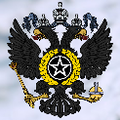# Corvette ship class Saburov

• 620 views, 0 today
• 32
• 9
• 4
Build by nagihihi <3 <3 <3 <3 <3 <3 <3 <3 <3 <3 <3 <3 <3 <3 <3 <3 <3 <3 <3 <3 <3 <3 <3 <3 <3 <3 < 3 <3 <3 <3 <3 <3 <3 <3 <3 <3 <3 <3 <3 <3 <3 <3 <3 <3 <3 <3 <3 <3 <3 <3 <3 <3 <3 <3 <3 <3 3 <3 <3 <3 <3 <3 <3 <3 <3 <3 <3 <3 <3 <3 <3 <3 <3 <3 <3 <3 <3 <3 <3 <3 <3 <3 <3 <3 <3 <3 3 <3 <3 <3 <3 <3 <3 <3 <3 <3 <3 <3 <3 <3 <3 <3 <3 <3 <3 <3 <3 <3 <3 <3 <3 <3 <3 <3 <3 <3 3 <3 <3 <3 <3 <3 <3 <3 <3 <3 <3 <3 <3 <3 <3 <3 <3 <3 <3 <3 <3 <3 <3 <3 <3 <3 <3 <3 < 3 <3 <3 <3 <3 <3 <3 <3 <3 <3 <3 <3
 Progress 100% complete Tags Water Structure

1
10/27/2021 2:25 pm
Level 18 : Journeyman Architect
Markus_INCYay it reached 30 diamonds!
1
10/28/2021 7:56 am
Level 63 : High Grandmaster Engineer
NagiFXup :D
1
10/19/2021 12:17 pm
Level 1 : New Miner
qwertyuiopoiytrewq